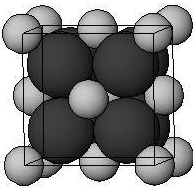Courses
Courses for Kids
Free study material
Free LIVE classes
MoreLIVE
Join Vedantu’s FREE Mastercalss

# Sodium oxide ${ Na }_{ 2 }{ O }$ is a crystalline solid, it has:A. Anti fluorite structureB. ${ Na }^{ + }$ ions are present at body diagonalsC. ${ Na }^{ + }$ ions are present at tetrahedral voidsD. ${ O }^{ 2- }$ ions are present at octahedral voidsVerified
333.9k+ views
Hint: A crystal or crystalline solid is a solid material whose constituent atoms, molecules, or ions are arranged in an orderly, repeating pattern extending in all three spatial dimensions. In crystallography, an anti-fluorite structure is acquired from a salt structure by exchanging anion and cation positions.

In the antifluorite structure, the anions adopt a face-centered cubic arrangement with cations in the tetrahedral interstices. The cations have a coordination number of 4 and the anions have a coordination number of 8.Figure: Cations are shown by black atoms and anions are shown by grey atoms.

In sodium oxide ${ Na }_{ 2 }{ O }$, ${ Na }^{ + }$ ions are present at tetrahedral voids and ${ O }^{ 2- }$ ions occupy half of the cubic holes in the face cubic close lattice. ${ Na }^{ + }$ ions are present at body diagonals.
${ Na }_{ 2 }{ O }$ is an anti fluorite structure.

Hence, the correct answer is option A,B,C.

The possibility to make a mistake is that you may not choose option B. But ${ Na }^{ + }$ ions are present at body diagonals, not on face diagonals.